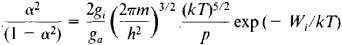# Saha Equation

The following article is from The Great Soviet Encyclopedia (1979). It might be outdated or ideologically biased.

## Saha Equation

a formula for the degree of thermal ionization α of a gas—that is, for the ratio of the number of ionized atoms to the total number of atoms present. First stated by M. Saha in 1920 for the description of processes in stellar atmospheres, the equation was derived from general thermodynamic considerations and deals with the case of a weakly ionized gas in a state of thermodynamic equilibrium. The equation has the formwhere T is the absolute temperature, p is the pressure of the gas, Wi is the ionization potential of the gas’ atoms, ga and gi are the statistical weights of the neutral atom and the ion, respectively, m is the mass of an electron, k is the Boltzmann constant, and h is Planck’s constant.

The Saha equation is only approximately valid because its derivation rests on the assumption that only three types of particles are present: neutral atoms, singly ionized ions, and electrons. Multiple ionization, excited atoms, and the presence of impurities are not taken into account. Also not considered is the interaction of the gas with the wall. Such interaction can result in surface ionization and in the ionization of the gas by electrons ejected from a hot wall. Despite these limitations, the Saha equation is applicable to many cases where α ≪ 1.

L. A. SENA

The Great Soviet Encyclopedia, 3rd Edition (1970-1979). © 2010 The Gale Group, Inc. All rights reserved.
References in periodicals archive ?
With these assumptions, the composition of plasma could be determined by solving the Saha equation. The Saha equation defines the relation of ions in two adjacent ionization stages.
For the simulation of capillary discharge used in the ET or ETC launch system, a problem of employing the method of Trayner and Glowacki or Zaghloul is that those conversation equations in the mathematical model of capillary discharge cannot give temperature directly whereas it is required as one of the inputting data pieces for solving the Saha equation. In the series researches of Zoler et al.
This method enables the work of solving the Saha equation to become rather simple in the simulation of capillary discharge; however, one still needs to solve an iteration equation with some numerical algorithm in each cell.
In the simulation research of capillary discharge for the ET or ETC launch technology, a widely used form of the Saha equation is given by 
Apparently, determining the composition of plasma by solving the Saha equation actually means determining [[psi].sub.ji] by solving (1).
Two supplementary equations for solving the Saha equation are given by
For solving the Saha equation expressed as (1), the density of plasma, whether the number density n or the mass density p, and T are required as the inputting data.
The method of solving the Saha equation individually listed below is from the work of Trayner and Glowacki and Zaghloul [12, 13]: for the element species j, (1) could be expressed as
Solving the Saha Equation in the Simulation of Capillary Discharge
Saha equation for two-temperature plasmas: Theories, experimental evidence, and interpretation.
An approach to a non-LTE Saha equation based on Druyvesteyn energy distribution function: A comparison between electron temperature obtained from OES and the Langmuir probe analysis.
In 1928, Milne would express concern relative to the appropriateness of the inferred thermal equilibrium in the reversing layer, as required by the Saha equations [36,37], although he believed that studies based on the validity of the ionization equations should be pursued: "The recent work of Adams and Russell brings forward evidence that the reversing layers of stars are not in thermodynamic equilibrium.

Site: Follow: Share:
Open / Close